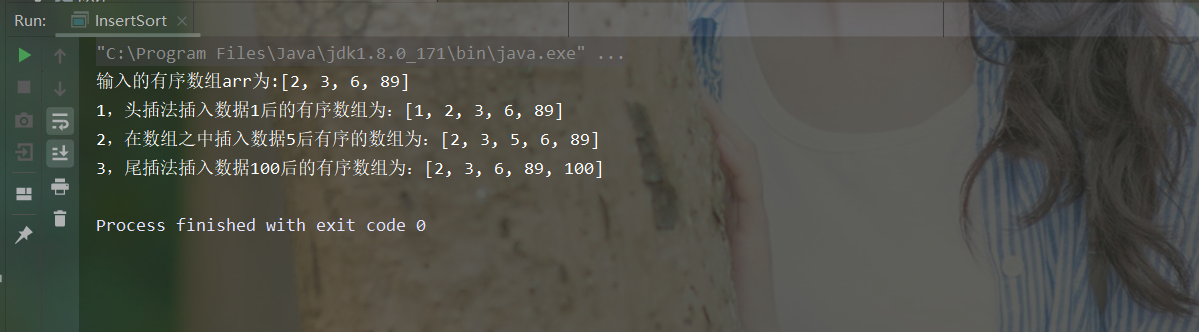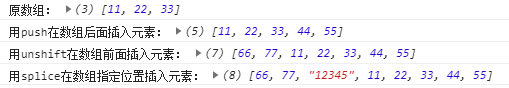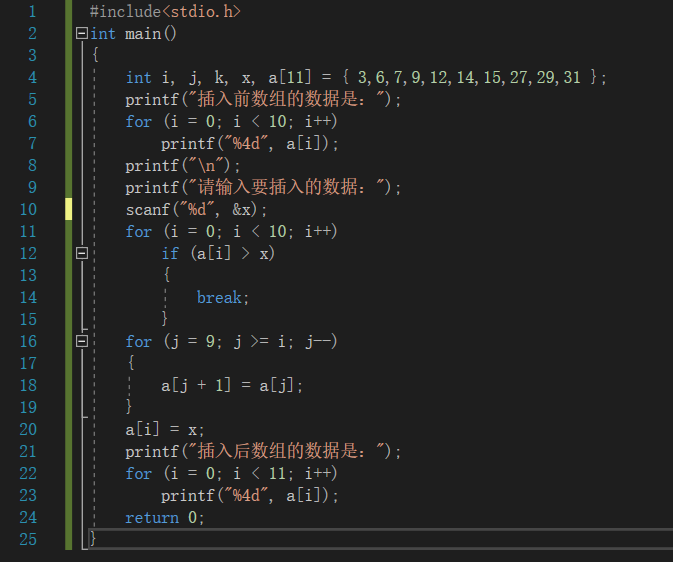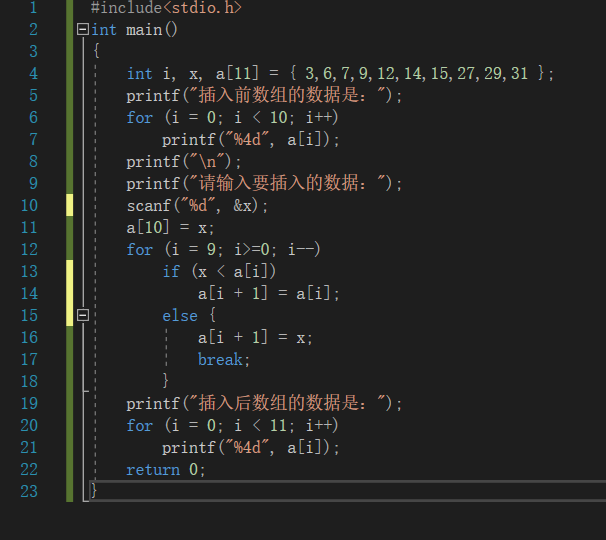• java数组实现针对一个有序的数组插入一数据并保持数组有序。
文章目录1，排序介绍2，思路分析3，java代码实现4，运行结果
1，排序介绍
针对这个问题，其实可以看做是插入排序中的有序排序。简单的介绍哈，插入排序的基本思想是将一个记录插入到已经排好序的有序表中，从而一个新的、记录数增1的有序表。
2，思路分析
可分为三种情况来考虑，第一种头插法也就是将数据插入到数组的第一个，这种情况插入的数据是最大值或最小值。第二种情况是将数据插入到数组中，插入的数组取值范围在最大值与最小值之间。第三种情况的也就是尾插法，插入的数据是最大值或最小值。
第一种头插法
输入有序数组：arr ={2,3,6,89}
插入的记录（数据）：1
输出插入数据的有序数组：arr ={1,2,3,6,89}
第二种插入数组之间
有序数组：arr ={2,3,6,89}
插入的记录（数据）：5
返回的有序数组：arr ={2,3,5,6,89}
第二种尾插法
有序数组：arr ={2,3,6,89}
插入的记录（数据）：100
返回的有序数组：arr ={2,3,6,89,100}
3，java代码实现
package sort;

import java.util.ArrayList;
import java.util.Arrays;
import java.util.List;

/**
* filename:  InsertSort
* IDE:   IntelliJ IDEA
* author: ganxiang
* CreateDate：2020-07-18 16:04
*/
public class InsertSort {

//头插法
private List insertHead(int [] arr,int data){
int len =arr.length;
int [] a =new int[len+1];
int k=0;
if (data<=arr)  a[k]=data;
for (int i = 0; i <=len-1 ; i++) {
k+=1;
a[k]=arr[i];
}
List<Integer> list =new ArrayList<>();
for (int num:a) {
}
return list;

}
//插入数据到数组之中
private List insertBody(int [] arr,int data){
int len =arr.length;
int [] a =new int[len+1];
int k=0;//通过移动k插入数据
for (int i = 0; i <=len-1 ; i++) {
if(data>=arr[i]&&data<=arr[i+1]){
a[k]=arr[i];
k+=1;
a[k]=data;
i+=1;
k+=1;
}
a[k]=arr[i];
k+=1;
}
//        System.out.println(Arrays.toString(a));
List<Integer> list =new ArrayList<>();
for (int num:a) {
}
return list;
}
//尾插法
private List insertTial(int [] arr,int data) {
int len = arr.length;
int[] a = new int[len + 1];
int k = 0;
for (int i = 0; i <= len - 1; i++) {
a[k] = arr[i];
k += 1;
}
if (data >= arr[len - 1]) a[k] = data;
List<Integer> list = new ArrayList<>();
for (int num : a) {
}
return list;
}

public static void main(String[] args) {
int [] arr ={2,3,6,89};
System.out.println("输入的有序数组arr为:"+Arrays.toString(arr));
System.out.println("2，在数组之中插入数据5后有序的数组为："+new InsertSort().insertBody(arr,5));
System.out.println("3，尾插法插入数据100后的有序数组为："+new InsertSort().insertTial(arr,100));
}

}

4，运行结果展开全文java 算法 排序算法
• ## php数组插入数据

千次阅读 2018-04-30 10:56:00
php数组插入数据 一、总结 代码要多敲，看是看不会的 php代码直接在页面不好敲，可以去控制器里面敲   二、目标 在一组数中，要求插入一个数，按其原来顺序插入，维护原来排序方式。   三、代码 （1）、...
php数组插入数据
一、总结
代码要多敲，看是看不会的
php代码直接在页面不好敲，可以去控制器里面敲

二、目标
在一组数中，要求插入一个数，按其原来顺序插入，维护原来排序方式。

三、代码
（1）、思路：找到比要插入数大的那个位置，替换，然后把后面的数后移一位。

1 <?php
2 $in = 2; 3$arr = array(1,1,1,3,5,7);
4 $n = count($arr);
5 //如果要插入的数已经最大，直接打印
6 if($arr[$n-1] < $in) { 7$arr[$n+1] =$in; print_r($arr); 8 } 9 for($i=0; $i<$n; $i++) { 10 //找出要插入的位置 11 if($arr[$i] >=$in){
12     $t1=$arr[$i]; 13$arr[$i] =$in;
14 //把后面的数据后移一位
15     for($j=$i+1; $j<$n+1; $j++) { 16$t2 = $arr[$j];
17       $arr[$j] = $t1; 18$t1 = $t2; 19 } 20 //打印 21 print_r($arr);
22   die;
23   }
24 }

1、print_r打印数组

（2）、

1 <!DOCTYPE html>
2 <html lang="en">
4     <meta charset="UTF-8">
5     <title>PHP Exercise</title>
7 <body>
8 <div>这是杨辉三角</div>
9     <?php
10         $in=2; 11$arr=array(1,1,1,3,5,7);
12         //1、将数组排序
13         sort($arr); 14 //2、二分查找在数组中找到要插入的位置 15$len=count($arr); 16$h=0;
17         $t=$len-1;
18         $in_pos=-1; 19 while($h<=$t){ 20$ave=intval(($h+$t)/2);
21             if($ave==$in){
22                 $in_pos=$ave;
23                 break;
24             }else if($ave>$in){
25                 $t=$ave-1;
26             }else{
27                 $h=$ave+1;
28             }
29         }
30         dump($in_pos); 31 //3、插入元素后面的数据依次后移,在数组中插入元素 32 ?> 33 </body> 34 </html> 1、sort()排序 2、count()函数计算元素数目 3、intval对浮点数取整 4、第22行，函数内可以直接用函数外的变量 5、php也支持break关键词 6、原生php也支持dump关键词  展开全文• push以及unshift即可向数组插入元素 如果要在指定的位置插入元素则可以用splice splice接收多个参数，分别是索引，要删除的元素个数，新加的元素(可多个，用逗号隔开)； let myArray=[11,22,33]; console.log('原...  push以及unshift即可向数组插入元素 如果要在指定的位置插入元素则可以用splice splice接收多个参数，分别是索引，要删除的元素个数，新加的元素(可多个，用逗号隔开)；  let myArray=[11,22,33]; console.log('原数组：',myArray); myArray.push(44,55); console.log('用push在数组后面插入元素：',myArray); myArray.unshift(66,77); console.log('用unshift在数组前面插入元素：',myArray); myArray.splice(2,0,'12345'); console.log('用splice在数组指定位置插入元素：',myArray);展开全文• C#定义个8位的维空数组，按下button0，插入数据"00"到第0位，替换之前的数据，按下button1，插入数据"01"到第1位，,,,,,,,,,一直到按下button7，发插入数据"07"到第7位。插入后替换掉数组之前的数据。。请问这段...c# • 请问插入和删除最后输出的那段的代码怎么写？拜托啦，急求！！！ #include #include struct Student { int num; char name; int score; struct Student*next; }; int main() { struct ...结构 • ## c语言数组中插入新数据 万次阅读 多人点赞 2019-01-03 15:05:06 数组插入数据 在数组的应用中，我们有时会向数组中插入一数据，而且不打破原来的排序规律，其实数组中的插入数据，就是数据的比较和移动；如果想要弄懂这些方法最好拿笔比划以下，或者debug一下，了解其中的... 数组插入数据 在数组的应用中，我们有时会向数组中插入一个数据，而且不打破原来的排序规律，其实数组中的插入数据，就是数据的比较和移动；如果想要弄懂这些方法最好拿笔比划以下，或者debug一下，了解其中的思想，光看理解的不深； 方法一： 输入一个数据x，将数组中的数据与x逐一比较，如果大于x，记录下数据的下标，然后此数据下标和其后的数据的下标都加一，相当于都向后挪一位，然后将x赋值给数组的那个下标；方法二： 第二种方法是将要插入的数据放在数组最后，然后和前面的数据逐一比较，如果x小于某元素a[i]，则将a[i]后移一个位置，否则将x至于a[i+1]的位置；展开全文• 返回目录 创建数组 var arr1 = new Array();//创建数组 var arr2 = new Array(size);//创建数组并指定长度，注意不是上限，是长度 var arr3 = new Array(element0, element1[, element3...插入数据 var arr =前端 • 现在整理一下Java中有序(假设是从小到大)数组插入数据的三种方式，其中种方式是直接调用static方法——Arrays.sort(数组名); 另外两种方法比较考验思维，都跟遍历有关。当然，除了这三种方法之外，还有许多其他的...java • JavaScript将数组插入到另个数组的方法。分享给大家供大家参考。具体分析如下： 1.通过Array.prototype.push.apply方法将数组插入到另外个数组，下面的代码将数组b插入到a var a = [2,3,4]; var b ... • 1. splice splice(position, ... item:插入的元素 如果只需要添加，不需要删除的话，我们可以封装个类似的函数（利用原型链） 原理：在原型链中增加个函数对象,删除的参数默认为0，如图 ... • 思路 1、将要插入的数据放到数组的最后一个位置 2、将新数据和前一个做比较，向前遍历 如果这个数据比前面一个小，就一直交换，一旦不小，就退出...//在有序数组插入一个元素 public class ArrayInsert { ...java基础 java数组 数组排序 • 我们知道数组是内存中一块连续的区域，一经开辟，不能随意调整大小， 借助Arrays.copyOf()函数可以快速调整数组大小，进而在数组尾部插入一数据java 算法 经验分享 程序人生 • 思路： 找要插入的位置 index 将该位置后面的数据，都往后挪一位 （处理index后面的数据） 把新数据插到该位置（处理index对应的... * 实现：在有序数组插入一个元素,保持数组仍然有序 * 对新数组排序---1）找...java基础 java数组 数组移动 • 数组添加到另数组中并去重，其中tableData是将要 添加到fatherTablelist的数组，这时建议用es6的reduce方法： inChildByValue: function(tableData){ if (this.fatherTablelist.leng... • ：Dart 向数组插入条数据。 二：Dart 向数组插入条数据 • 数组算法 往数组尾部添加一条数据1202 for (int i = 0; i < 数组名.length; i++) { if (数组名[i] == null) { 数组名[i] = 要插入的数据; break; } } • 1、在默认的情况下，Vue.js默认不支持往数组中...2、不使用track-by="$index"的数值插入数组不支持重复数据插入  2.1 JavaScript代码 window.onload = function() { vm = new Vue({ el: '#app',Vue.js Vue
• var arr1 = ['a', 'b', 'c'];...// 把arr2 变成个适合splice的数组（包含splice前2个参数的数组） arr2.unshift(2, 0); console.log(arr2); //[2, 0, "1", "2", "3"] Array.prototype.splice.app...
• 方法1var arr1 = ['a', '...// 把splice的前2个参数插入到arr2的前面 arr2.unshift(2, 0); // 或者先把arr2 reverse 翻转，再把参数push进去，然后再把数组翻转回来 console.log(arr2); //[2, 0, "1", "2", "3"] ...
• array(22) { ["user_name"]=> array(4) { => string(3) "name1" => string(3) "name2" => string(3) "name3" ...这是4条数据,请问大神们这怎么插入数据库呢?新手求教!!!/(ㄒoㄒ)/~~
• 今天闲着无聊测试了一下js数组splice方法的性能发现：当数组的长度大于100000的时候整个页面会出于比较长的卡死状态，故试着写了个性能更好的方法来实现批量在数组头部插入数据： let splice = function (arr) { ...
• 这里写自定义目录标题欢迎使用Markdown编辑器新的改变功能快捷键合理的创建标题，有助于目录的生成如何改变文本的样式插入链接与图片如何插入一段漂亮的代码片生成一个适合你的列表创建一个表格设定内容居中、居左、...前端 asp.net微信小程序 微信小程序
• * 在数组指定位置插入数组 * @param array $array 原数组 * @param int$position 需要插入到原数组的位置 * @param array $insert_array 需要插入数组 * @return array */ function designatPosInsertArr(&...php 后端 • insert into t_RolePermission( [f_intRoleId] ,[f_intPermissionId] ,[f_strNote] ) select 109 as roleId, f_intPermissionId as permissionId, null as notefrom t_Permission where f_intPerminsert delete null • var ary1 = [1, 2, 4, 5, 7, 8,10,11]; var ary2 = [3, 6, 9,12]; for (let i = 0; i < ary2.length; i++) { ary1.splice(2 + 3 * i, 0, ary2[i]); } console.log(ary1... • 如果没有移除任何值，则此数组中的元素将插入到指定位置。 案例、$a1=array(0=>"Dog",1=>"Cat",2=>"Horse",3=>"Bird"); $a2=array(0=>"Tiger",1=>"Lion"); array_splice($a1,0,2,$a2); print_r($a1...php
• public class ListInsert { public static long[] insert(long[] arr,int i,long l){ //新建数组,对原数组扩容 long[] arr1 = new long[arr.... //将原数组数据赋值给新数组 for(int j = 0;j;j++){ arr1[j]
• // 要插入的位置 NSIndexSet*helpIndex = [NSIndexSetindexSetWithIndexesInRange:NSMakeRange(1, [helpSellArrcount])];...// 要插入数组 [self.helpArr insertObjects:helpSellArr atIndexes:helpIndex]; /...